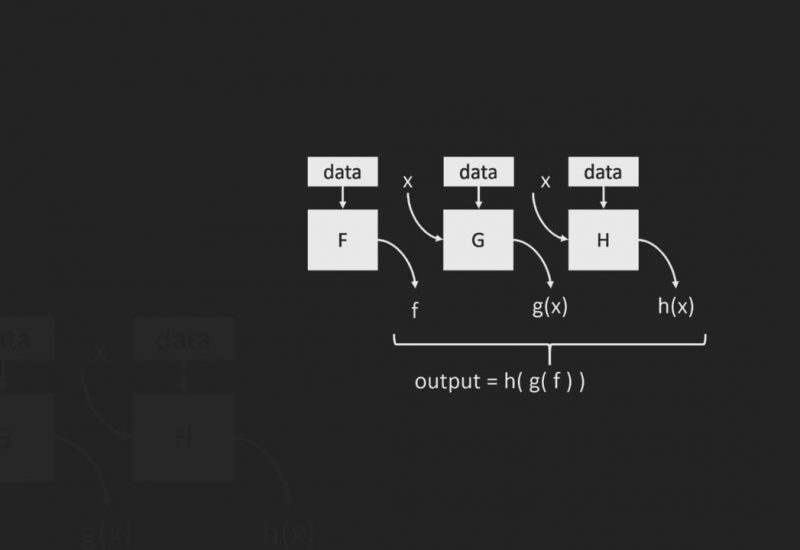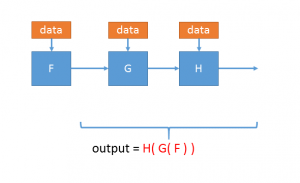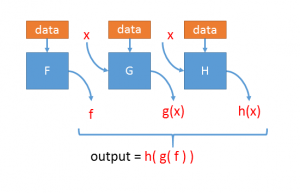Established: October 17, 2014

## Overview

Project Parade is a novel approach to parallelizing a large class of seemingly sequential applications wherein dependencies are, at runtime, treated as symbolic values. The efficiency of parallelization, then, depends on the efficiency of the symbolic computation, an active area of research in static analysis, verification, and partial evaluation. This is exciting as advances in these fields can translate to novel parallel algorithms for sequential computation.

#### Project Parade in the News

Here’s an example of how it works. Imagine an algorithm that has three components, F, G, and H. F takes some data as input and generates a result. That result is used by G, possibly along with some other data, to compute its result. Then H uses G’s result (and again, possibly some other data) to compute the final result.Because of these dependencies, G cannot start executing until F has finished. Likewise, H cannot start executing until G has finished. How can we possibly execute this algorithm in parallel? We do that by starting G (and H) at the same time as F. F executes using the real input data, but G and H are given a symbolic input, x. They are then executed in a symbolic manner which generates a summary: g(x) for G and h(x) for H. A summary is itself a function that, given a concrete input, generates a concrete (i.e., not symbolic) output. So once F has computed its output, that is used by g(x) to compute the output that is then used as input by h(x).The final, concrete, result is the same as that computed by the sequential algorithm. In order for this to be efficient, it must be possible to do two things:

1. The summary of a component must be “small”: i.e., it must be concise enough so that it can be communicated easily in a parallel implementation to the process that will execute it on concrete input.
2. The execution of a summary must be efficient (which is related to its size): if it takes as long to execute the summary as it does to execute the original component, then the parallel implementation will be no faster than the sequential algorithm.

## Symbolic Execution

Here’s an example of how it works. Imagine an algorithm that has three components, F, G, and H. F takes some data as input and generates a result. That result is used by G, possibly along with some other data, to compute its result. Then H uses G’s result (and again, possibly some other data) to compute the final result.Because of these dependencies, G cannot start executing until F has finished. Likewise, H cannot start executing until G has finished. How can we possibly execute this algorithm in parallel? We do that by starting G (and H) at the same time as F. F executes using the real input data, but G and H are given a symbolic input, x. They are then executed in a symbolic manner which generates a summary: g(x) for G and h(x) for H. A summary is itself a function that, given a concrete input, generates a concrete (i.e., not symbolic) output. So once F has computed its output, that is used by g(x) to compute the output that is then used as input by h(x).The final, concrete, result is the same as that computed by the sequential algorithm. In order for this to be efficient, it must be possible to do two things:

1. The summary of a component must be “small”: i.e., it must be concise enough so that it can be communicated easily in a parallel implementation to the process that will execute it on concrete input.
2. The execution of a summary must be efficient (which is related to its size): if it takes as long to execute the summary as it does to execute the original component, then the parallel implementation will be no faster than the sequential algorithm.